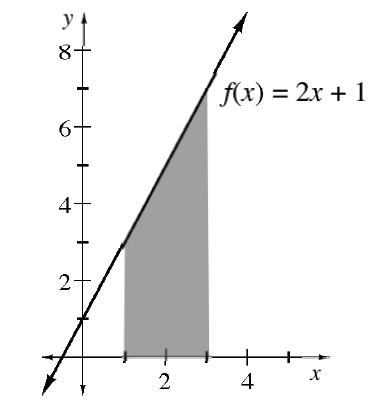### Home > APCALC > Chapter 4 > Lesson 4.1.3 > Problem4-33

4-33.

Given the graph at right of $f\left(x\right) = 2x + 1$, evaluate:

1. $\int _ { 1 } ^ { 3 } ( 2 x + 1 ) d x$

It's a trapezoid!

2. $\int _ { 1 } ^ { 3 } ( 2 t + 1 ) d t$

You could sketch another graph where the horizontal axis is called $t$. Or you could let $x = t$ and use the graph above.

3. What is the difference between the expressions in parts (a) and (b)?

None. The finite areas are the same.